# Intel景深攝影機Python程式詳解2-於NVIDIA Jetson Nano執行RealSense D435 範例

*本文由RS components 贊助發表，轉載自DesignSpark部落格原文連結

 作者/攝影 張嘉鈞 難度 ★★☆☆☆（普通） 材料表 NVIDIA Jestson Nano Developer Kit PC or Notebook INTEL RealSense D435 RK-NVIDIA® Jetson Nano™ Developer Kit B01 套件

# 目錄

• 對齊RGB跟Depth畫面並去除背景 ( align-depth2color.py )
• 解決掉幀問題 ( frame_queue_example.py )
• 結語

# 對齊RGB跟Depth畫面並去除背景 ( align-depth2color.py )

``# 導入函式庫import pyrealsense2 as rsimport numpy as npimport cv2# 建立一個context物件存放所有 RealSense 的處理函示pipeline = rs.pipeline()# 配置串流物件config = rs.config()``

``# 可以透過下列程式取得設備資訊# 透過 resolve 確認第一個可用的設備pipeline_wrapper = rs.pipeline_wrapper(pipeline)pipeline_profile = config.resolve(pipeline_wrapper)# 取得該設備資訊device = pipeline_profile.get_device()device_product_line = str(device.get_info(rs.camera_info.product_line))# 建立「深度」影像串流config.enable_stream(rs.stream.depth, 640, 480, rs.format.z16, 30) # uint 16# 建立「彩色」影像串流# 由於 L500 系列的彩色攝影機解析度維 960 x 540 所以才需要取得設備資訊if device_product_line == 'L500':    config.enable_stream(rs.stream.color, 960, 540, rs.format.bgr8, 30)else:    config.enable_stream(rs.stream.color, 640, 480, rs.format.bgr8, 30)# 開啟影像串流profile = pipeline.start(config)``

``# 取得深度感測器物件depth_sensor = profile.get_device().first_depth_sensor()# 取得深度感測器的 深度資訊與實際公尺之間的 映射尺度 # get_depth_scale -> Retrieves mapping between the units of the depth image and metersdepth_scale = depth_sensor.get_depth_scale()print("Depth Scale is: " , depth_scale)# 宣告多少公尺以內的資訊要顯示clipping_distance_in_meters = 1 #1 meter# 將 公尺資訊 轉換成 RealSense 的 深度單位 clipping_distance = clipping_distance_in_meters / depth_scale``

``# 建立 對齊 物件 ( 以 color 為基準 )align_to = rs.stream.color# rs.align 讓 深度圖像 跟 其他圖像 對齊align = rs.align(align_to)# 使用 process 來實現剛剛宣告的 align 對齊功能# aligned_frames = align.process(frames)``

``# 串流迴圈try:    while True:                # 等待最新的影像，wait_for_frames返回的是一個合成的影像        frames = pipeline.wait_for_frames()        # frames.get_depth_frame() is a 640x360 depth image        # 使用 process 來實現剛剛宣告的 align 對齊功能        aligned_frames = align.process(frames)``

``# 取得對齊後的影像        depth_frame = frames.get_depth_frame()        aligned_depth_frame = aligned_frames.get_depth_frame() # aligned_depth_frame is a 640x480 depth image        color_frame = aligned_frames.get_color_frame()        # 驗證是否都有影像        if not aligned_depth_frame or not color_frame:            continue        # 轉換成 numpy         depth_image = np.asanyarray(aligned_depth_frame.get_data())        color_image = np.asanyarray(color_frame.get_data())        # 顯示解析度        # print('Org (L500) : ', np.shape(np.asanyarray(depth_frame.get_data())), end='\t')        # print('After align : ', np.shape(np.asanyarray(aligned_depth_frame.get_data()))) ``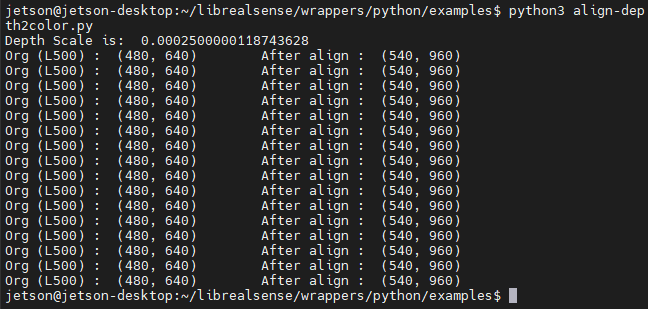``# 刪除背景 : 將背景顏色調整成 灰色         grey_color = 153        # 深度影像只有 1 個 channel，透過 dstack 建立 灰階圖 ( 這邊RGB的數值就是 深度訊息 )        depth_image_3d = np.dstack((depth_image, depth_image, depth_image)) #depth image is 1 channel, color is 3 channels            # 當 深度大於 1 公尺 或是 偵測不出來 就會 以 grey_color 取代        bg_removed = np.where((depth_image_3d > clipping_distance) | (depth_image_3d <= 0), grey_color, color_image)``

``# 將深度影像轉換成 彩色影像        depth_colormap = cv2.applyColorMap(cv2.convertScaleAbs(depth_image, alpha=0.03), cv2.COLORMAP_JET)        # 合併圖像 左為去背圖，右為深度圖        images = np.hstack((bg_removed, depth_colormap))        # cv2.namedWindow('Align Example', cv2.WINDOW_NORMAL)        cv2.imshow('Align Example', images)        key = cv2.waitKey(1)        # Press esc or 'q' to close the image window        if key & 0xFF == ord('q') or key == 27:            cv2.destroyAllWindows()            breakfinally:    pipeline.stop()``

``import numpy as npa = np.asanyarray( [[8000, 2000],                    [0, 125]])b = np.dstack((a,a,a))print(a.shape, b.shape)print('\n')print(a)print('\n')print(b) ``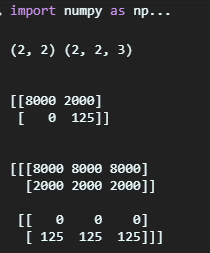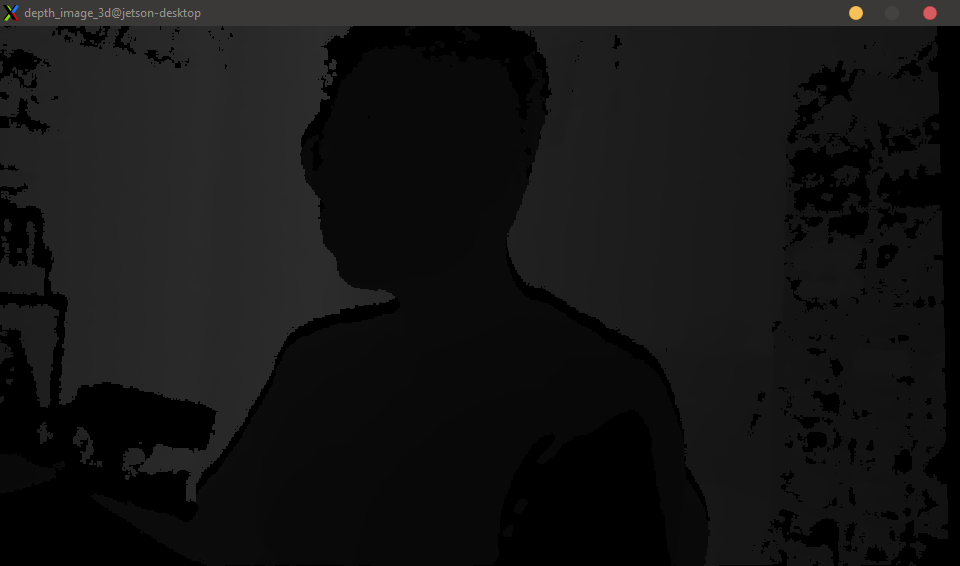# 解決掉幀問題 ( frame_queue_example.py )

``# First import the libraryimport pyrealsense2 as rsimport time# 每20個frame會暫停0.25秒 製造短暫的延遲def slow_processing(frame):    n = frame.get_frame_number()     if n % 20 == 0:        time.sleep(1/4)    print(n)# 製造更延遲的狀況def slower_processing(frame):    n = frame.get_frame_number()     if n % 20 == 0:        time.sleep(1)    print(n)``

``try:    # Create a pipeline    pipeline = rs.pipeline()    # Create a config and configure the pipeline to stream    #  different resolutions of color and depth streams    config = rs.config()    config.enable_stream(rs.stream.depth, 640, 480, rs.format.z16, 30)``

``   print("Slow callback")    # 開始影像串流    pipeline.start(config)    # 讓 slow_processing 運行5秒就好    start = time.time()    while time.time() - start < 5:        # 取得最新的影像物件        frames = pipeline.wait_for_frames()        # 運行slow_processing        slow_processing(frames)    # 關閉RealSense物件    pipeline.stop()``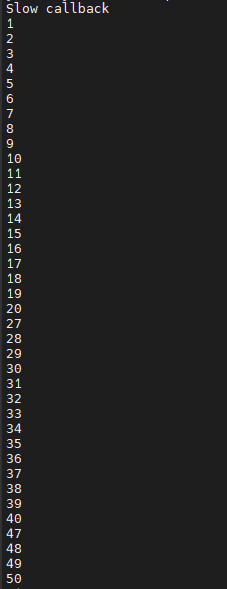``# 加上 queue     print("Slow callback + queue")    # frame_queue(n) n 是可以保留多少幀，這邊保留了50幀的空間    queue = rs.frame_queue(50)    # 開啟串流    pipeline.start(config, queue)    # 執行五秒    start = time.time()    while time.time() - start < 5:        frames = queue.wait_for_frame()        # 一樣使用 slow_processing        slow_processing(frames)``

``    print("Slower callback + queue")    queue = rs.frame_queue(50)    pipeline.start(config, queue)    start = time.time()    while time.time() - start < 5:        frames = queue.wait_for_frame()        slower_processing(frames)    pipeline.stop()``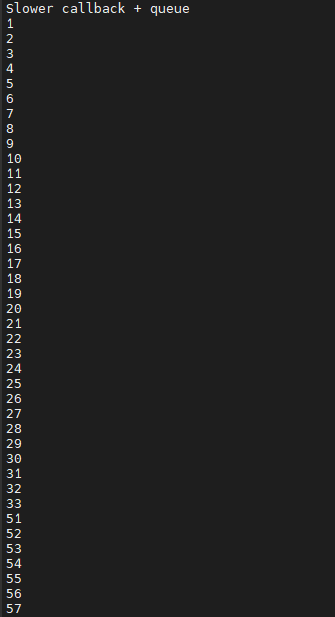``    print("Slower callback + keeping queue")    queue = rs.frame_queue(50, keep_frames=True)    pipeline.start(config, queue)    start = time.time()    while time.time() - start < 5:        frames = queue.wait_for_frame()        slower_processing(frames)    pipeline.stop()``

# 錄製深度影像 (.bag)

``# 建立一個context物件存放所有 RealSense 的處理函示pipeline = rs.pipeline()# 配置串流物件config = rs.config()# 建立「深度」影像串流config.enable_stream(rs.stream.depth, 640, 480, rs.format.z16, 30)# 將影像數據輸出到 test.bag 當中config.enable_record_to_file('test.bag')``

``import pyrealsense2 as rsimport numpy as npimport cv2import argparseimport os.pathtry:    pipeline = rs.pipeline()    config = rs.config()    # 告訴 config 資訊從檔案讀取    rs.config.enable_device_from_file(config, 'test.bag')    # 一樣要建立深度影像串流    config.enable_stream(rs.stream.depth, rs.format.z16, 30)    # 開啟串流    pipeline.start(config)    # 宣告著色器 預設是 jet colormap    colorizer = rs.colorizer();``

``    while True:                frames = pipeline.wait_for_frames()        depth_frame = frames.get_depth_frame()        # 將深度影像著色成 jet colormap        depth_color_frame = colorizer.colorize(depth_frame)        # 轉換成 numpy array 讓 opencv 顯示        depth_color_image = np.asanyarray(depth_color_frame.get_data())        # Opencv 顯示以及按下 q 離開        cv2.imshow("Depth Stream", depth_color_image)        key = cv2.waitKey(1)        if key == 27:            cv2.destroyAllWindows()            breakfinally:    pass``

# 結語

*本文由RS components 贊助發表，轉載自DesignSpark部落格原文連結